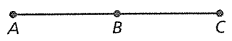Mathematics
Easy

Question

# Four steps of a proof are shown.Given: AC = AB + ABProve: AB = BCWhat is step no. 3? Statements Reason 1. AC = AB + AB 1. Given 2. AB + BC = AC   3. AB + AB = AB + BC   4. AB = BC

## Substitution Property of EqualityTransitive propertySegment Addition PostulateSubtraction property of equalityHint:

## The correct answer is: Substitution Property of Equality

### Given AB + AB = ACAB + BC = ACSo , using Substitution Property of EqualityThe substitution property of equality states that if two quantities are equal, then one can replace the other in any Mathematical equation or expression.AB + AB = AB + AC

The substitution property of equality states that if two quantities are equal, then one can replace the other in any Mathematical equation or expression.

### Related Questions to study#### With Turito Foundation.#### Get an Expert Advice From Turito.